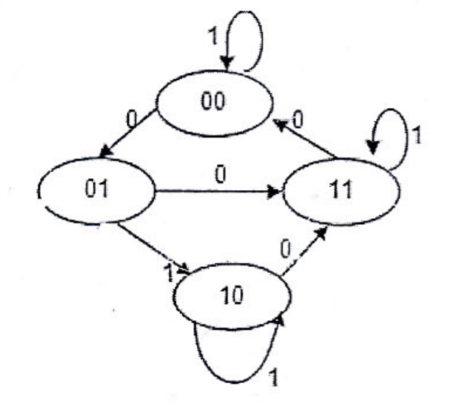### Exam Year

Tribhuvan University

Institute of Science and Technology

2077

Bachelor Level / first-semester / Science

Computer Science and Information Technology( CSC111 )

Digital Logic

Full Marks: 60 + 20 + 20

Pass Marks: 24 + 8 + 8

Time: 3 Hours

Candidates are required to give their answers in their own words as far as practicable.

The figures in the margin indicate full marks.

Group A

Attempt any two questions (2 x 10 = 20)

1

Design a combinational circuit that generates 9’s complement of a BCD number.

2

Implement the following functions using PLA

1. w (A, B, C, D) = Σ(2, 12, 13)
2. x (A, B, C, D) = Σ(7, 8, 9, 10, 11, 12, 13, 14, 15)
3. y (A, B, C, D) = Σ(0, 2, 3, 4, 5, 6, 7, 8, 9, 10, 11, 15)
4. z (A, B, C, D) = Σ(1, 2, 8, 12, 13)

3

Design sequential circuit specified by the following state diagram using T flip-flops.Group B

Attempt any eight questions (8 x 5 = 40)

4

List two major characteristics of digital computer. Represent -6 (negative six) 8 bits in signed magnitude, signed 1’s complement and signed 2’s complement respectively. Represent decimal number 4673 in a) octal and b) BCD

5

Where is CMOS suitable to use? Define Power dissipation. Show that the positive logic NAND gate is a negative logic NOR gate and vice versa.

6

Simplify the following function and implement them with two level NOR gate circuit, F(w,  x,  y,  z) = wx’ + y’z’ + w’yz’.

7

Design a full subtractor circuit with three inputs x, y, $$B_{in}$$ and two outputs Diff and $$B_{out}$$· The circuit subtracts $$x-y-B_{in}$$., where $$B_{in}$$ is the input borrow, $$B_{out}$$ is the output borrow, and Diff is the difference.

8

Design 4-bit even parity generator.

9

What is the difference between a serial and parallel transfer? Explain how to convert serial data to parallel and parallel data to serial. What type of register is needed?

10

Explain negative-edge triggered D flip flop with necessary logic diagram and truth table.

11

Illustrate the use of Binary ripple counter and BCD ripple counter.

12

Write Short notes on (Any two)

1. RTL
2. State Reduction
3. POS